Check the below NCERT MCQ Questions for Class 10 Maths Chapter 6 Triangles with Answers Pdf free download. MCQ Questions for Class 10 Maths with Answers were prepared based on the latest exam pattern. We have provided Triangles Class 10 Maths MCQs Questions with Answers to help students understand the concept very well. https://ncertmcq.com/mcq-questions-for-class-10-maths-with-answers/

Students can also refer to NCERT Solutions for Class 10 Maths Chapter 6 Triangles for better exam preparation and score more marks.

## Triangles Class 10 MCQs Questions with Answers

Triangles Class 10 MCQ Question 1.
In the given figure, ∠T and ∠B are right angles. If the length of AT, BC and AS (in centimeters) are 15, 16, and 17 respectively, then the length of TC (in centimeters) is: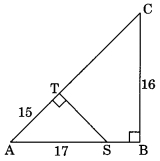(a) 18
(b) 16
(c) 19
(d) 12

Class 10 Triangles MCQ Question 2.
In the given figure, value of x(in cm) is(a) 4
(b) 5
(c) 6
(d) 8

Triangles MCQ Class 10 Question 3.
In the given figure ΔABC ~ ΔPQR. The value of x is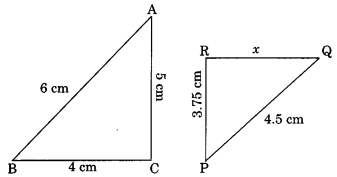(a) 2.5 cm
(b) 3.5 cm
(c) 2.75 cm
(d) 3 cm

Triangle Class 10 MCQ Question 4.
In ΔABC, if DE || BC, AD = x, DB = x – 2, AE = x + 2 and EC = x – 1, then value of x is
(a) 3
(b) 4
(c) 5
(d) 3.5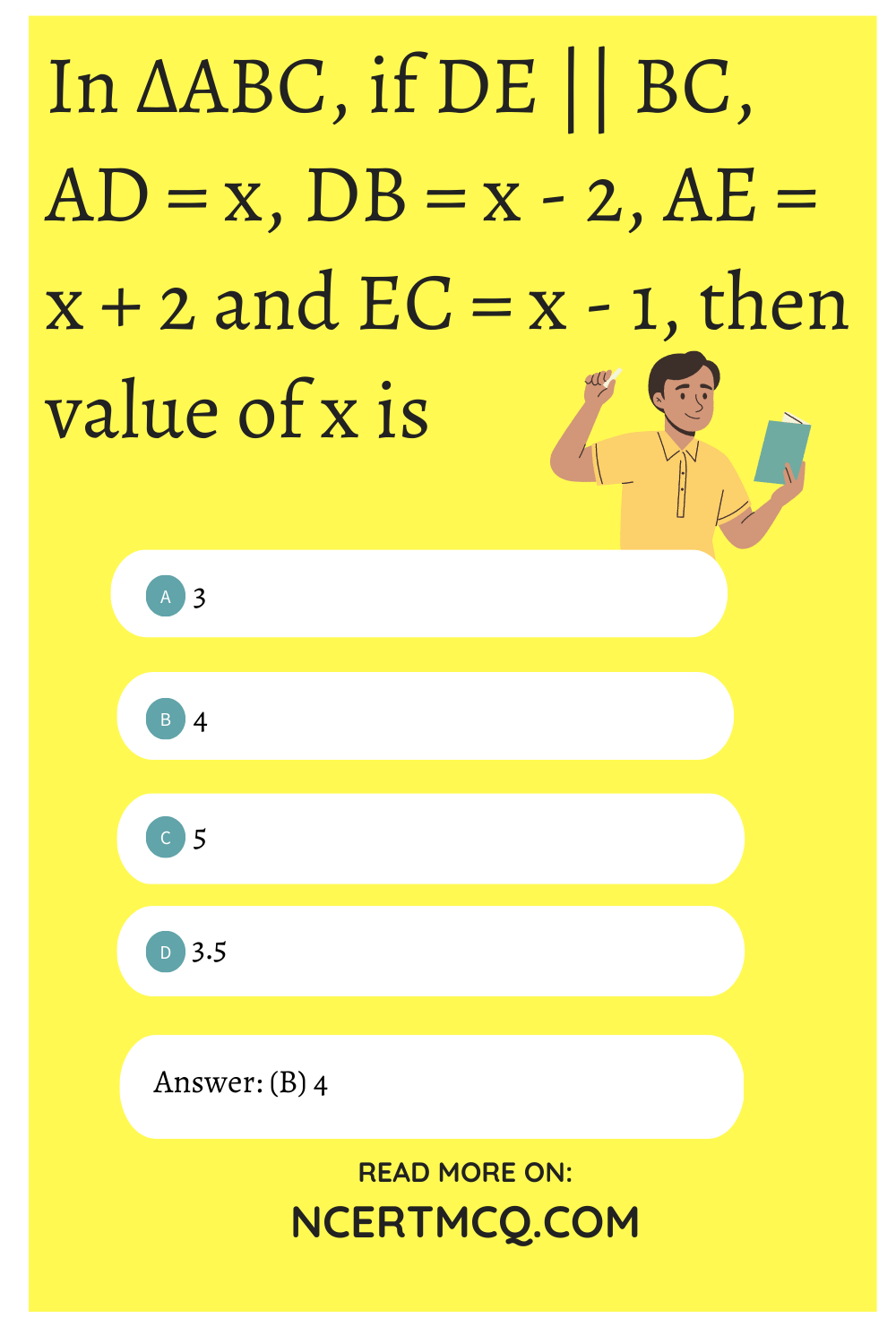Triangle MCQ Class 10 Question 5.
The perimeters of two similar triangles ABC and PQR are 60 cm and 36 cm respectively. If PQ = 9 cm, then AB equals
(a) 6 cm
(b) 10 cm
(c) 15 cm
(d) 24 cm

MCQ On Triangles Class 10 Question 6.
If ΔABC is similar to ΔDEF such that 2 AB = DE and BC = 8 cm then EF is equal to.
(a) 12 cm
(b) 4 cm
(c) 16 cm
(d) 8 c

Triangles Class 10 MCQ With Answers Question 7.
In ΔABC, AB = 6 cm and DE || BC such that AE = $$\frac{1}{4}$$ AC then the length of AD is
(a) 2 cm
(b) 1.2 cm
(c) 1.5 cm
(d) 4 cm

MCQ Of Triangles Class 10 Question 8.
In the given figure DE || AC which of the following is true?(a) x = $$\frac{a+b}{ay}$$
(b) y = $$\frac{ax}{a+b}$$
(c) x = $$\frac{ay}{a+b}$$
(d) $$\frac{x}{y}$$ = $$\frac{a}{b}$$

Answer: (c) x = $$\frac{ay}{a+b}$$

Class 10 Maths Chapter 6 MCQ Question 9.
ΔABC ~ ΔDEF. If AB = 4 cm, BC = 3.5 cm, CA = 2.5 cm and DF = 7.5 cm, then the perimeter of ΔDEF is
(a) 10 cm
(b) 14 cm
(c) 30 cm
(d) 25 cm

MCQ Triangles Class 10 Question 10.
In the figure PQ || BC. If $$\frac{PQ}{BC}$$ = $$\frac{2}{5}$$ then $$\frac{AP}{PB}$$ is(a) $$\frac{2}{5}$$
(b) $$\frac{2}{3}$$
(c) $$\frac{3}{2}$$
(c) $$\frac{3}{5}$$

Answer: (b) $$\frac{2}{3}$$

Class 10 Triangle MCQ Question 11.
In the given figure, ΔACB ~ ΔAPQ. If AB = 6 cm, BC = 8 cm, and PQ = 4 cm then AQ is equal to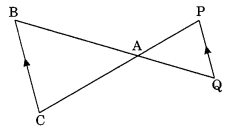(a) 2 cm
(b) 2.5 cm
(c) 3 cm
(d) 3.5 cm

Ch 6 Maths Class 10 MCQ Question 12.
ΔDEF ~ ΔABC. If DE : AB = 2 : 3 and ar ΔDEF is equal to 44 square units then ar (ΔABC) (square unit) is
(a) 99
(b) 120
(c) $$\frac{176}{9}$$
(d) 66

MCQs On Triangles Class 10 Question 13.
ΔABC and ΔBDE are two equilateral triangles such that D is the mid point of BC. Ratio of the areas of triangle ΔABC and ΔBDE is.
(a) 2 : 1
(b) 1 : 2
(c) 4 : 1
(d) 1 : 4

Chapter 6 Maths Class 10 MCQ Question 14.
If ΔABC ~ ΔPQR, $$\frac{ar ΔABC}{ar ΔPQR}$$ = $$\frac{9}{4}$$ and AB = 18 cm, then the length of PQ is
(a) 14 cm
(b) 8 cm
(c) 10 cm
(d) 12 cm

Class 10 Maths Ch 6 MCQ Question 15.
In the given figure ΔABC ~ ΔPQR, PM is median of ΔPQR. If ar ΔABC = 289 cm², BC = 17 cm, MR = 6.5 cm then the area of ΔPQM is(a) 169 cm²
(b) 13 cm²
(c) 84.5 cm²
(d) 144.5 cm²

Question 16.
If the ratio of the perimeters of two similar triangles is 4 : 25, then the ratio of the areas of the similar triangles is
(a) 16 : 625
(b) 2 : 5
(c) 5 : 2
(d) 625 : 16

Question 17.
In the given figure, PQ = 24 cm, QR = 26 cm ∠PAR = 90°, PA = 6 cm, and AR = 8 cm, the degree measure of ∠QPR is(a) 90°
(b) 100°
(c) 50°
(d) 45°

Question 18.
In the given figure the value of x is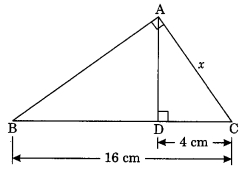(a) 4 cm
(b) 5 cm
(c) 8 cm
(d) 3 cm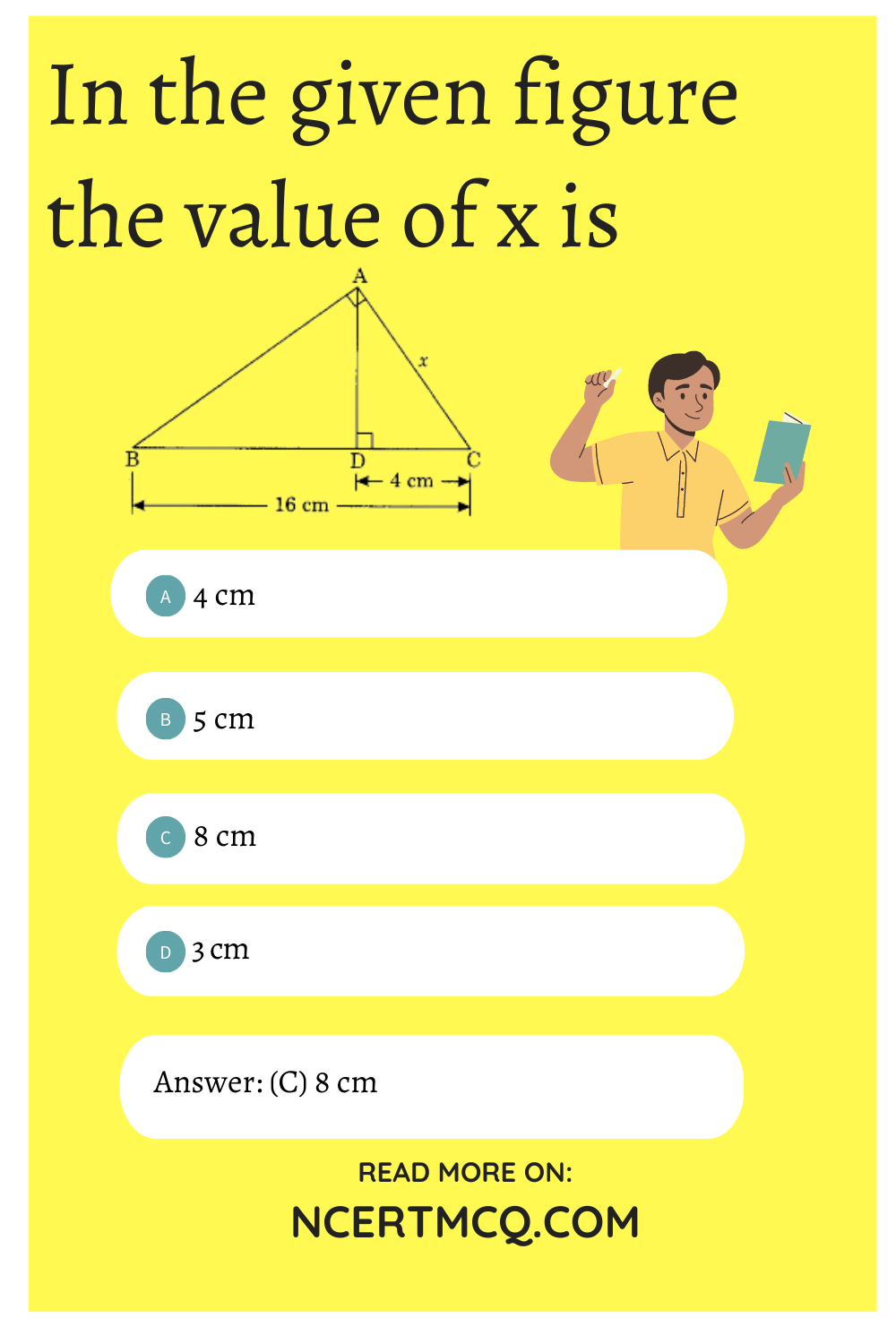Question 19.
ΔPQR is an equilateral triangle with each side of length 2p. If PS ⊥ QR, then PS is equal to
(a) $$\frac{√3}{2}$$p
(b) 2p
(c) √3p
(d) p

Question 20.
In ΔLMN, ∠L = 50° and ∠N = 60°, If ΔLMN ~ ΔPQR, then find ∠Q
(a) 50°
(b) 70°
(c) 60°
(d) 40°